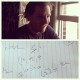# Laruelle: from identity to inclusion

I think of Laruelle’s notion of identity as working a bit like the way inclusion functions work (with some caveats, which I’ll raise at the end). Here’s how.

The identity function on a domain is the function which sends every value in that domain to itself: f(x) = x.

Every function has a domain (which identifies the kinds of values it accepts as inputs) and a codomain (which identifies the kinds of values it produces as outputs). We can write this as follows: f : A -> B means “f has the domain A, and the codomain B”, or “f accepts values of type A, and produces values of type B”. The identity function IdA : A -> A is the function f(x) = x for the domain A; that is, it accepts values from the domain A as inputs, and send every value in that domain to itself. Similarly, there is an identity function IdB: B -> B which is the function f(x) = x for the domain B; and so on.

An inclusion function from a domain A to a codomain B, where A is a subset of B, is almost exactly like the identity function on A – it sends every value in A to itself – except that the codomain is B rather than A. So it is the function f : A -> B where f(x) = x.

For example, there is an inclusion function from Z, the set of integers, to R, the set of real numbers, which just is the identity function on Z except that the codomain is R rather than Z. It sends every integer to itself, but to itself “considered as” a real number (since the integers are a subset of the reals). We might say that every integer has a “regional” identity as an integer-among-integers, mapped by the identity function IdZ : Z -> Z, and an “integer-among-the-reals” identity as a real number, mapped by the inclusion function f : Z -> R. Both functions are identical in terms of their inputs and outputs, but they have different meanings.

Now, it seems to me that for Laruelle, the Real is “like” (but see caveats below) a sort of global codomain that absolutely everything has an inclusion function into, since all “regional” domains are just subsets of this codomain. So for absolutely anything you like, it has both a “regional” identity (mapped by the identity function on its regional domain) and a One-in-one identity (mapped by the inclusion function from that domain into the Real).

When we consider everything in the aspect of its One-in-one identity, we can consider juxtapositions that aren’t otherwise possible. For example, let M be the domain of men, and F the domain of women. If we know that there is a common codomain (H, the domain of human beings) of which both M and F are subsets, then we can consider the identities man-among-humans and woman-among-humans as potentially overlapping, able to be combined or mutually transformed in various ways that the identities man-among-men and woman-among-women seemingly are not.

The Laruellian principle of unilateral identity-in-the-last-instance with the Real can be understood as something “like” a global generalisation of this move from identity to inclusion.

Now, there are two caveats to be raised here. The first is that, in set theoretic terms, a global codomain is impossible, because of Russell’s paradox – “the One is not”, as Badiou says. Accordingly, we have to pass from something like a set into something like a “proper class”; and functions are defined between sets, not between sets and proper classes. So at the threshold of the Real, the mathematical analogy breaks down, as we should probably expect it to.

The second is that Laruelle himself is quite emphatic that the kinds of ordering and partitioning operations that sets and functions between sets enable you to perform, belong to the domain of “transcendental” material – language, symbolisation, the material through which the force-of-thought has its material effects. The real, being One, is non-partitionable; and, being foreclosed to thought, is not indexable or schematisable in the ways that the set-theoretic mathematical universe is. (The move to the “quantum” is I think intended as a move outside the set-theoretic mathematical universe; I’ll talk a bit more about that some other time). “Regional” domains may be structured and more-or-less set-like, but that is both their prerogative and their weakness with respect to the Real (or its weakness with respect to them, since it underdetermines them). We must not picture whatever structures we can imagine being stabilised, held fixedly within an underlying global order of structure that is just like them only somehow bigger.

The problem then is that a global generalisation of the move from identity to inclusion takes us beyond what is structurally thinkable, or at least beyond what is thinkable using the tools we use to think about and within regional domains. This prevents us from setting up any particular regional domain (e.g. “physics”) as a master-domain against which all the others can be relativised. We have in Laruelle something like an anti-totalitarian thinking of (or “according to”) totality.

## One thought on “Laruelle: from identity to inclusion”

1.jdempcy says:

Fascinating article. I was just reading Louis Kauffman’s work on eigenforms and self-referential propositions whose terms “re-enter their own indicational space.” These are, at their simplest, precisely expressed as x = f(x). We can step through it to see what he means by re-entering indicational space…

x = f(x)
x = f(f(x))
x = f(f(f(x)))
x = f(…)

We can verify in our imagination that this recursively repeats ad infinitum. Kauffman calls this “re-entering the indicational space” but also understands it in other ways. Since most of Kauffman’s work is way over my head, I will defer to his essay here, for a better explanation!

http://www.gwu.edu/~rpsol/preconf/wmsci/kaufman2.pdf